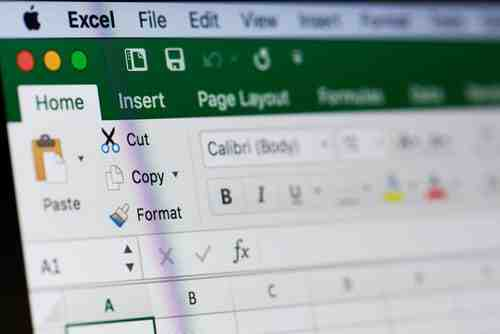Latest update on February 12, 2021 at 11:59 AM by Natalia Kudryavtseva .Microsoft Excel gives users the flexibility to configure data and perform very basic operations, such as addition, subtraction, multiplication, or division by using shortcuts or custom formulas.This article will introduce some of the most basic functions and formulas offered by Microsoft Excel. While seemingly simplistic, mastering the use of these formulas and functions is the foundation for all other complex functionalities available on the office software.

Basic Formulas on Microsoft Excel

It's important to note that all formulas must be preceded by the = sign. If the symbol is not found, Microsoft Excel will recognize the entry as plain text.

If you'd like to find the sum of your data data in rows A1 to A10 in your spreadsheet, you may do so by using the following formula:

=SUM(A1: A10)

If you are looking to add data in cells that are not juxtaposed, you may do so by simply typing =SUM and clicking directly on the cells you'd like to include. Make sure that the cells listed are surrounded by parenthesis (i =SUM(A1, A3; C4)).

Average Formula

If you'd like to find the average of your data in rows A1 to A10 in your spreadsheet, you may do so by using the following formula:

=Average(A1: A10)

Maximum and Minimum Formula

These two formulas may be used to find the maximum and minimum numbers of data points located in rows A1 to A10 in your spreadsheet:

=Max(A1: A10)

=MIN(A1: A10)

The IF Function

The IF function may be used to apply a condition to your data. For example, if a manager would like to be notified when stock on a product reaches zero, he may use this function to program a custom notification to appear in an adjacent cell. This sort of formula would look like this:

IF(A1 <= 0; "to order", "in stock")

In this case, if the contents of cell A1 is less than or equal to zero, the words "to order" will appear in an adjacent cell. If the contents of cell A1 is greater than zero, the column will read "in stock."

A more general IF function would look like this:

= IF (condition; value "if true"; value "otherwise")

Freeze Cells in Excel

Freezing cells allows you to keep an area of your worksheet visible when you scroll to another area of the page. It's important to note that you can only freeze rows at the top of your worksheet and columns on the left side of your worksheet. You cannot freeze rows and columns in the middle.

To freeze specific tabs, head to the View tab. Here, you have the option to either Freeze Top Row or Freeze Top Column. Click either option to lock your cells in place.

If you'd like to lock multiple rows, simply select the row below the last row you'd like to freeze and then head to the View tab > Freeze Panes. Note that all rows up to and including row one will be locked.

To freeze multiple columns, highlight the column to the right of the last column that you'd like to freeze, and then head to the View tab > Freeze Panes. Note that all columns up to and including column A will be locked.

How to Create Custom Excel Functions?

To create a custom function on Excel, you will need to use the VBA programming language, but don’t worry, you don’t have to be a programmer to do it. Read our article about Macros in Excel and how to work in the Developer mode to create your own functions and automate them in Excel.

Image: © Dzmitry Kliapitski - Shutterstockom

##### Tags: